Next: 1-d problem with mixed Up: The diffusion equation Previous: The diffusion equation

## Introduction

The diffusion equation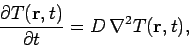(187)

where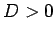is the (uniform) coefficient of diffusion, describes many interesting physical phenomena. For instance, in heat conduction we can write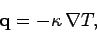(188)

where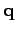is the heat flux,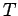the temperature, and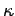the coefficient of thermal conductivity. The above equation merely states that heat flows down a temperature gradient. In the absence of sinks or sources of heat, energy conservation requires that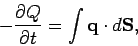(189)

where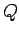is the thermal energy contained in some volumebounded by a closed surface. The above equation states that the rate of decrease of the thermal energy content of volumeequals the instantaneous heat flux flowing across its boundary. However,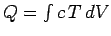, where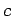is the heat capacity per unit volume. Making use of the previous equations, as well as the divergence theorem, we obtain the following diffusion equation for the temperature: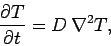(190)

where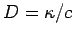. In a typical heat conduction problem, we are given the temperature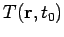at some initial time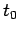, and then asked to evaluate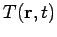at all subsequent times. Such a problem is known as an initial value problem. The spatial boundary conditions can be either of type Dirichlet (i.e.,specified on the boundary), type Neumann (i.e.,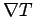specified on the boundary), or some combination.Next: 1-d problem with mixed Up: The diffusion equation Previous: The diffusion equation
Richard Fitzpatrick 2006-03-29